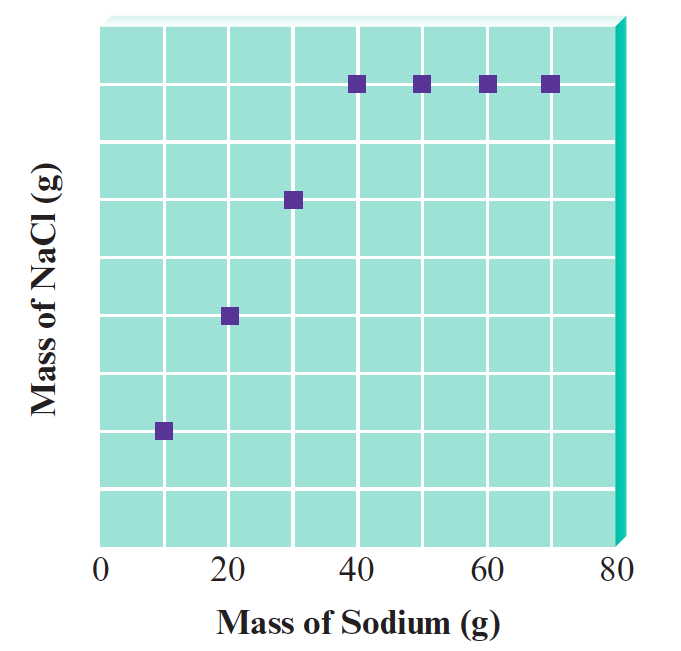# Problem: You have seven closed containers, each with equal masses of chlorine gas (Cl  2). You add 10.0 g of sodium to the first sample, 20.0 g of sodium to the second sample, and so on (adding 70.0 g of sodium to the seventh sample). Sodium and chlorine react to form sodium chloride according to the equation2 Na (s) + Cl2 (g) → 2 NaCl (s)After each reaction is complete, you collect and measure the amount of sodium chloride formed. A graph of your results is shown below.Calculate the mass of NaCl formed when 50.0 g of sodium is used.

###### FREE Expert Solution

We’re being asked to calculate the mass of NaCl formed when 50.0 g of sodium is used. We're given the following balanced reaction:

2 Na (s) + Cl2 (g) → 2 NaCl (s)

We don't know the amount of Cl2 used but we can calculate it based on the graph given. Each container has equal masses of chlorine gas (Cl2). We will use the part of the graph where the amount of NaCl produced becomes constant. This means that all Cl2 is used up.

• some amount of Cl2 produced 80 g of NaCl
• from the balanced reaction: 1 mole of Cl2 produces 2 moles of NaCl
• molar mass of NaCl = 58.44 g/mol
• molar mass of Cl2 = 70.9 g/mol

90% (172 ratings)###### Problem Details

You have seven closed containers, each with equal masses of chlorine gas (Cl  2). You add 10.0 g of sodium to the first sample, 20.0 g of sodium to the second sample, and so on (adding 70.0 g of sodium to the seventh sample). Sodium and chlorine react to form sodium chloride according to the equation

2 Na (s) + Cl2 (g) → 2 NaCl (s)

After each reaction is complete, you collect and measure the amount of sodium chloride formed. A graph of your results is shown below.Calculate the mass of NaCl formed when 50.0 g of sodium is used.

Frequently Asked Questions

What scientific concept do you need to know in order to solve this problem?

Our tutors have indicated that to solve this problem you will need to apply the Limiting Reagent concept. You can view video lessons to learn Limiting Reagent. Or if you need more Limiting Reagent practice, you can also practice Limiting Reagent practice problems.

What professor is this problem relevant for?

Based on our data, we think this problem is relevant for Professor Fadeev's class at SHU.

What textbook is this problem found in?

Our data indicates that this problem or a close variation was asked in Chemistry: An Atoms First Approach - Zumdahl Atoms 1st 2nd Edition. You can also practice Chemistry: An Atoms First Approach - Zumdahl Atoms 1st 2nd Edition practice problems.